Courses

# 28 Year NEET Questions: Some Basic Concepts Of Chemistry - 1

## 18 Questions MCQ Test Chemistry 31 Years NEET Chapterwise Solved Papers | 28 Year NEET Questions: Some Basic Concepts Of Chemistry - 1

Description
This mock test of 28 Year NEET Questions: Some Basic Concepts Of Chemistry - 1 for NEET helps you for every NEET entrance exam. This contains 18 Multiple Choice Questions for NEET 28 Year NEET Questions: Some Basic Concepts Of Chemistry - 1 (mcq) to study with solutions a complete question bank. The solved questions answers in this 28 Year NEET Questions: Some Basic Concepts Of Chemistry - 1 quiz give you a good mix of easy questions and tough questions. NEET students definitely take this 28 Year NEET Questions: Some Basic Concepts Of Chemistry - 1 exercise for a better result in the exam. You can find other 28 Year NEET Questions: Some Basic Concepts Of Chemistry - 1 extra questions, long questions & short questions for NEET on EduRev as well by searching above.
QUESTION: 1

### In Haber process 30 litres of dihydrogen and 30 litres of dinitrogen were taken for reaction which yielded only 50% of the expected product. What will be the composition of gaseous mixture under the aforesaid condition in the end? 

Solution: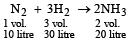It is given that only 50% of the expected product is formed.
Hence, only 10 litre of NH3 is formed i.e. N2 used = 5 litres ⇒ N2 left = 30 – 5 = 25 litres
H2 used = 15 litres ⇒ H2 left = 30 – 15 = 15 litres

QUESTION: 2

### The maximum number of molecules is present in:

Solution:

No. of molecules in different cases:

(a) 22.4 litre at STP contains = 6.023×1023 molecule of H2
∴ 15 litre at STP containsmolecules

(b) 22.4 litre at STP contains = 6.023×1023 molecule of N2
∴ 5 litre at STP contains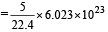molecules

(c) 2 gm of  H= 6.023×1023 molecules of  H2
∴ 0.5 gm of H2 contains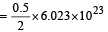molecules

(d) Similarly, 10 g of O2 gas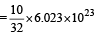molecules

Thus (a) will have maximum number of molecules

QUESTION: 3

### The mass of carbon anode consumed (givin g only carbondioxide) in the production of 270 kg of aluminium metal from bauxite by the Hall process is  (Atomic mass: Al = 27) 

Solution:

2Al2O3 + 3C → Al3CO2

Gram equivalent  of Al2O3 = Gram equivalent of C

Equivalent weight of Al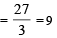Equivalent weight of C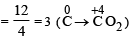No. of gram equivalent of Al  =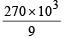= 30 × 103
Hence, No. of gram equivalent of C = 30 × 103

Again, No. of gram equivalent of C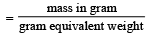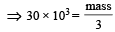⇒  mass = 90 × 10g = 90 kg

QUESTION: 4

The number of moles of KMnO4 reduced by one mole of KI in alkaline medium is:   

Solution: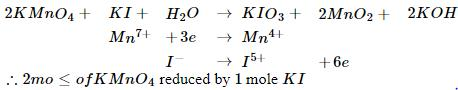QUESTION: 5

Concentrated aqueous sulphuric acid is 98% H2SO4 by mass and has a density of 1.80 g mL– 1. Volume of acid required to make one litre of 0.1MH2SO4 solution is 

Solution:

Molarity of H2SO4 solution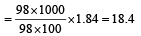Suppose V ml of this H2SO4 is used to prepare 1 lit. of 0.1M H2SO4
∴ V x 18.02 = 1000 x 0.1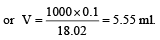QUESTION: 6

An element , X has the following isotopic composition :
200X:90 %
199X : 8.0%
202X ; 2.0 %
The weighted average atomic mass of the naturally occuring element X is closest to

Solution:

Average isotopic mass of,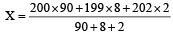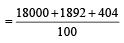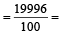199.96 amu

QUESTION: 7

The number of moles of KMnO4 that will be needed to react with one mole of sulphite ion in acidic solution is 

Solution:

The balanced chemical equation is: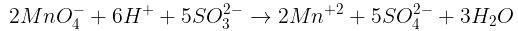From the equation, it is clear that 2 moles of MnO4  are required to oxidize 5 moles of SO3–2
2/5 mole of MnO4 is required to oxidize 1 mole of SO3–2

QUESTION: 8

Volume occupied by one molecule of water (density = 1 g cm–3) is : 

Solution: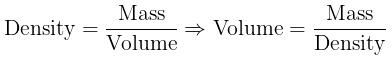Density of water = 1 gram cm–3
∴ Volume occupied by 1 gram water = 1 cm3
i.e. Volume occupied by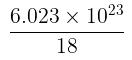molecules of water = 1 cm3 [∵ 1g water = 1/18moles of water]

Thus volume occupied by 1 molecule of water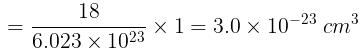QUESTION: 9

Number of moles of MnO4- required to oxidize one mole of ferrous oxalate completely in acidic medium will be : 

Solution: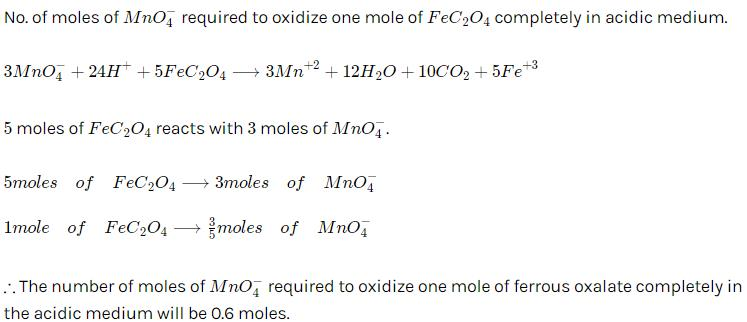QUESTION: 10

What volume of oxygen gas (O2) measured at 0°C and 1 atm, is needed to burn completely 1L of propane gas (C3H8) measured under the same conditions? 

Solution:

Writing the equation of  combustion of propane (C3H8), we get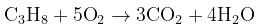From the above equation we find that we need 5 L of oxygen at NTP to completely burn 1 L of propane at N.T.P.
If we change the conditions for both the gases from N.T.P. to same conditions of temperature and pressure. The same results are obtained. i.e. 5 L is the correct answer.

QUESTION: 11

An organic compound contains carbon, hydrogen and oxygen. Its elemental analysis gave C, 38.71% and H, 9.67%. The empirical formula of the compound would be: 

Solution: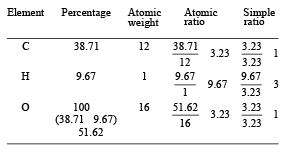Thus empirical formula is CH3O.

QUESTION: 12

How many moles of lead (II) chloride will be formed from a reaction between 6.5 g of PbO and 3.2 g of HCl ? 

Solution:

Writing the equation for the reaction, we get: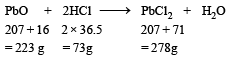From this equation, we find 223 g of PbO reacts with 73 g of HCl to form 278 g of PbCl2.
If we carry out the reaction between 3.2 g HCl and 6.5 g PbO.
Amount of PbO that reacts with 3.2 g HCl =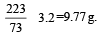Since the amount of PbO present is only 6.5 g so PbO is the limiting reagent.

Amount of PbCl2 formed by 6.5 g of PbO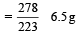Number of moles of PbCl2 formed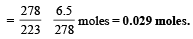QUESTION: 13

10 g of hydrogen and 64 g of oxygen were filled in a steel vessel and exploded. Amount of water produced in this reaction will be:

Solution: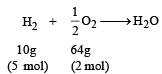In this reaction, oxygen is the limiting agent.
Hence the amount of H2O produced depends on the amount of O2 taken
0.5 mole of O2 gives H2O = 1 mol
∴ 2 mole of O2 gives H2O = 4 mol

QUESTION: 14

What is the [OH] in the final solution prepared by mixing 20.0 mL of 0.050 M HCl with 30.0 mL of 0.10 M Ba(OH)2? 

Solution:

No. of milli equivalent of HCl = 20 × 0.05 = 1.0
No. of milli equivalent of Br (OH)2   = 30 × 0.1 × 2 = 6.0
After neutralization, no. of milli equivalents in 50 ml. of solution = (6 – 1) = 5
Total volume of the solution = 20 + 30 = 50 ml
∴ No. of milli equivalent of OHis 5 in 50 ml,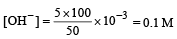QUESTION: 15

The number of atoms in 0.1 mole of a triatomic gas is:  (NA = 6.02 ×1023 mol–1)

Solution:

The number of atoms in 0.1 mole of a triatomic gas = 0.1 × 3 × 6.023 × 1023.= 1.806 × 1023

QUESTION: 16

Which has the maximum number of molecules among the following ? [2011 M]

Solution: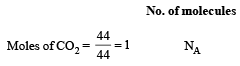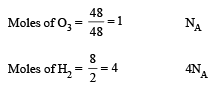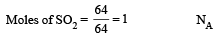Hence, option C is correct.

QUESTION: 17

6.02 × 1020 molecules of urea are present in 100 mL of its solution. The concentration of solution is: [NEET 2013]

Solution: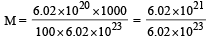QUESTION: 18

In an experiment it showed that 10 mL of 0.05 M solution of chloride required 10 mL of 0.1 M solution of AgNO3, which of the following will be the formula of the chloride (X stands for the symbol of the element other than chlorine): [NEET Kar. 2013]

Solution:

Millimoles of solution of chloride = 0.05 × 10 = 0.5
Millimoles of AgNO3 solution = 10 × 0.1 = 1
So, the millimoles of AgNO3 are double than the chloride solution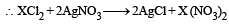Track your progress, build streaks, highlight & save important lessons and more!

### Similar Content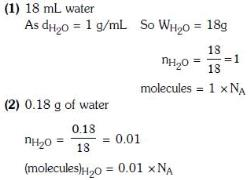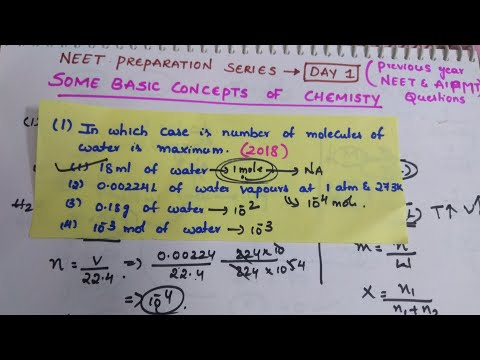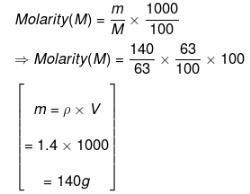### Related tests